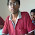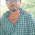# Pharma Engineering

An Engineer's Choice

• ## Sunday, 27 March 2016

Got a query asking How to Calculate the volume occupied by torispherical dish of a batch reactor,
this query will definitely have a solution, but usually many engineers will keep following some of the thumb rules those are applicable here, and some of the others will apply whatever thumb they remember, so its better to use thumb rule practically, but before that its very recommend to know the reason behind deriving the thumb.

So before going into calculation directly, first of all we should know some basic concepts involved in the design of torispherical dish [doom/disc].,

** Crown RadiusA torispherical dish is the surface obtained from the intersection of a spherical cap with a tangent torus, as Shown in above pic. The radius of the sphere R is called the "crown radius,"

** Knuckle Radius: The Radius of the circle's those are formed at the transition phase from spherical surface to flat surface is called Knuckle Radius, Usually the sphere's / circle's those were formed at those transition phases were called Torus, and in plural form mentioned as Torii, so having torii is the reason behind deriving the name as torispherical dishes, And

generally the knucle radius of a torispherical dish will be 0.6 times the diameter of the vessel.

Usually these torispherical dishes were designed for the sake of pressure vessels and storage vessels, to avoid the breathing losses in such vessels these dishes were designed.

You May Also Like:

** Critical Radius: The Radius where the transition occurs from Spherical to Torus is called as Critical Radius.,

So, if you are clear with the above basic definitions we can enter into calculation part,

Letbe the distance from the center of the torus to the center of the torus tube, let 'c' be the radius of the torus tube, and let 'h' be the height from the base of the dome to the top. Then the radius of the base is given by a + c < R. In addition, by elementary geometry, a torispherical dome satisfies

The transition from sphere to torus occurs at the critical radius

So from the basic Dome equation from 3d Co-ordinate geometry, we are having two equations based on different cases

 The Torispherical Dome has VolumeSo, these are the theoretical Myth that our thumb is basing , and now i'm gonna tell you the thumb for calculating the Torispherical end volume,

Volume occupied by torispherical dish, V= (Pi/24)* Di*Di*Di.,

And some people want to have some clarity even through Thumb, so for them practice this,

Volume, V= [0.0847*Di*Di*Di]+ [ (pi*Di*Di*S.F)/4].

Thats it......Cheers,

Some of the Readers wont have much time to read and digest all the above theory and may need instant results, for them i have developed an excel sheet, use that sheet for calculation,

You May Also Like:Hi! I am Ajay Kumar Kalva, Currently serving as the CEO of this site, a tech geek by passion, and a chemical process engineer by profession, i'm interested in writing articles regarding technology, hacking and pharma technology.

1.Dear Ajay, here you given the volume occupied by the torispherical dish pi/24*di^3, is it only for one dish or two dishes? noramally reactor have two dishes. And what is the meaning of S.F below this eqation?

1.It is the single dish tentative volume, S.F. means height of straight flange.

Regards,
AJAY K

2.1.3.what is Di

1.Internal diaHi! I am Ajay Kumar Kalva, Currently serving as the CEO of this site, a tech geek by passion, and a chemical process engineer by profession, i'm interested in writing articles regarding technology, hacking and pharma technology.